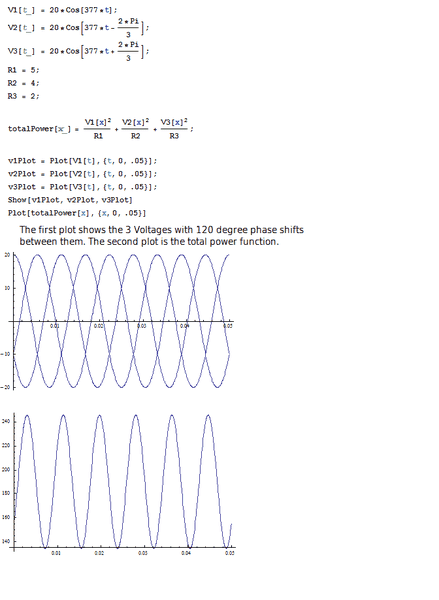# 3 Phase Electric Power Question

I have a question and am wondering if reddit can help. In a 3 phase power system, the overall total power is meant to remain constant. Now, I understand that the individual powers are meant to vary, but as a system, it is supposed to be a constant. If you could take a look at this "code" if you wish that I've created in Mathematica, it is apparent that the power is time varying. I must be doing something wrong, any help?jim hardy
Gold Member
Dearly Missed
....but as a system, it is supposed to be a constant.

Are you sure that statement is true for unbalanced system?

I know nothing of 'Mathematica" , but suggest you test your code by setting all three resistors equal...

Are you sure that statement is true for unbalanced system?

I know nothing of 'Mathematica" , but suggest you test your code by setting all three resistors equal...

I see. You sir are correct. I completely forgot about the fact that three different line impedances would put the system out of balance. I set the impedance values to be equal and of course, the power remained constant.

Thanks a lot Jim!